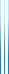# PublicationsManuscripts
Berge (A theorem related to the Chvátal conjecture) showed that if $\mathcal{F}$ is a downwards-closed family of sets, then either $\mathcal{F}$ or $\mathcal{F} \setminus \{\emptyset\}$ can be partitioned into pairs of disjoint sets.